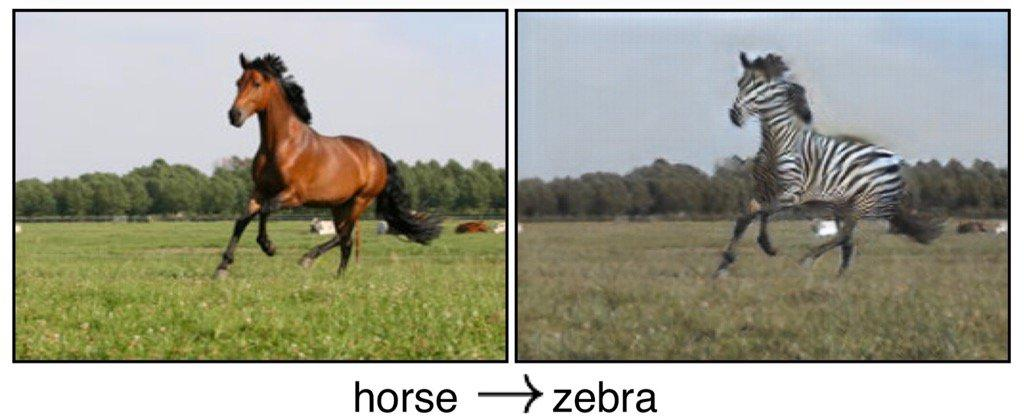@王小新 编译自 GitHub

（文末有风格迁移小demo）

[1703.10593] Unpaired Image-to-Image Translation using Cycle-Consistent Adversarial Networks

# 构建生成器

``````ngf = 32 # Number of filters in first layer of generator
ndf = 64 # Number of filters in first layer of discriminator
batch_size = 1 # batch_size
pool_size = 50 # pool_size
img_width = 256 # Imput image will of width 256
img_height = 256 # Input image will be of height 256
img_depth = 3 # RGB format``````

``````o_c1 = general_conv2d(input_gen,
num_features=ngf,
window_width=7,
window_height=7,
stride_width=1,
stride_height=1)``````

``````def general_conv2d(inputconv, o_d=64, f_h=7, f_w=7, s_h=1, s_w=1):
with tf.variable_scope(name):
conv = tf.contrib.layers.conv2d(inputconv, num_features, [window_width, window_height], [stride_width, stride_height],
biases_initializer=tf.constant_initializer(0.0))``````

``````o_c2 = general_conv2d(o_c1, num_features=64*2, window_width=3, window_height=3, stride_width=2, stride_height=2)
# o_c2.shape = (128, 128, 128)

o_enc_A = general_conv2d(o_c2, num_features=64*4, window_width=3, window_height=3, stride_width=2, stride_height=2)
# o_enc_A.shape = (64, 64, 256)``````

``````o_r1 = build_resnet_block(o_enc_A, num_features=64*4)
o_r2 = build_resnet_block(o_r1, num_features=64*4)
o_r3 = build_resnet_block(o_r2, num_features=64*4)
o_r4 = build_resnet_block(o_r3, num_features=64*4)
o_r5 = build_resnet_block(o_r4, num_features=64*4)
o_enc_B = build_resnet_block(o_r5, num_features=64*4)

# o_enc_B.shape = (64, 64, 256)
``````

Resnet模块的代码如下：

``````def resnet_blocks(input_res, num_features):

out_res_1 = general_conv2d(input_res, num_features,
window_width=3,
window_heigth=3,
stride_width=1,
stride_heigth=1)
out_res_2 = general_conv2d(out_res_1, num_features,
window_width=3,
window_heigth=3,
stride_width=1,
stride_heigth=1)
return (out_res_2 + input_res)``````

``````o_d1 = general_deconv2d(o_enc_B, num_features=ngf*2 window_width=3, window_height=3, stride_width=2, stride_height=2)
o_d2 = general_deconv2d(o_d1, num_features=ngf, window_width=3, window_height=3, stride_width=2, stride_height=2)``````

``gen_B = general_conv2d(o_d2, num_features=3, window_width=7, window_height=7, stride_width=1, stride_height=1)``

``````def build_generator(input_gen):
o_c1 = general_conv2d(input_gen, num_features=ngf, window_width=7, window_height=7, stride_width=1, stride_height=1)
o_c2 = general_conv2d(o_c1, num_features=ngf*2, window_width=3, window_height=3, stride_width=2, stride_height=2)
o_enc_A = general_conv2d(o_c2, num_features=ngf*4, window_width=3, window_height=3, stride_width=2, stride_height=2)

# Transformation
o_r1 = build_resnet_block(o_enc_A, num_features=64*4)
o_r2 = build_resnet_block(o_r1, num_features=64*4)
o_r3 = build_resnet_block(o_r2, num_features=64*4)
o_r4 = build_resnet_block(o_r3, num_features=64*4)
o_r5 = build_resnet_block(o_r4, num_features=64*4)
o_enc_B = build_resnet_block(o_r5, num_features=64*4)

#Decoding
o_d1 = general_deconv2d(o_enc_B, num_features=ngf*2 window_width=3, window_height=3, stride_width=2, stride_height=2)
o_d2 = general_deconv2d(o_d1, num_features=ngf, window_width=3, window_height=3, stride_width=2, stride_height=2)
gen_B = general_conv2d(o_d2, num_features=3, window_width=7, window_height=7, stride_width=1, stride_height=1)

return gen_B
``````

``````o_c1 = general_conv2d(input_disc, ndf, f, f, 2, 2)
o_c2 = general_conv2d(o_c1, ndf*2, f, f, 2, 2)
o_enc_A = general_conv2d(o_c2, ndf*4, f, f, 2, 2)
o_c4 = general_conv2d(o_enc_A, ndf*8, f, f, 2, 2)``````

``decision = general_conv2d(o_c4, 1, f, f, 1, 1, 0.02)``

``````input_A = tf.placeholder(tf.float32, [batch_size, img_width, img_height, img_layer], name="input_A")
input_B = tf.placeholder(tf.float32, [batch_size, img_width, img_height, img_layer], name="input_B")``````

``````gen_B = build_generator(input_A, name="generator_AtoB")
gen_A = build_generator(input_B, name="generator_BtoA")
dec_A = build_discriminator(input_A, name="discriminator_A")
dec_B = build_discriminator(input_B, name="discriminator_B")

dec_gen_A = build_discriminator(gen_A, "discriminator_A")
dec_gen_B = build_discriminator(gen_B, "discriminator_B")
cyc_A = build_generator(gen_B, "generator_BtoA")
cyc_B = build_generator(gen_A, "generator_AtoB")``````

1. 鉴别器必须允许所有相应类别的原始图像，即对应输出置1；

2. 鉴别器必须拒绝所有想要愚弄过关的生成图像，即对应输出置0；

3. 生成器必须使鉴别器允许通过所有的生成图像，来实现愚弄操作；

4. 所生成的图像必须保留有原始图像的特性，所以如果我们使用生成器GeneratorA→B生成一张假图像，那么要能够使用另一个生成器GeneratorB→A来努力恢复成原始图像。此过程必须满足循环一致性。

``````D_A_loss_1 = tf.reduce_mean(tf.squared_difference(dec_A,1))
D_B_loss_1 = tf.reduce_mean(tf.squared_difference(dec_B,1))``````

``````D_A_loss_2 = tf.reduce_mean(tf.square(dec_gen_A))
D_B_loss_2 = tf.reduce_mean(tf.square(dec_gen_B))

D_A_loss = (D_A_loss_1 + D_A_loss_2)/2
D_B_loss = (D_B_loss_1 + D_B_loss_2)/2``````

``````g_loss_B_1 = tf.reduce_mean(tf.squared_difference(dec_gen_A,1))
g_loss_A_1 = tf.reduce_mean(tf.squared_difference(dec_gen_A,1))``````

``cyc_loss = tf.reduce_mean(tf.abs(input_A-cyc_A)) + tf.reduce_mean(tf.abs(input_B-cyc_B))``

``````g_loss_A = g_loss_A_1 + 10*cyc_loss
g_loss_B = g_loss_B_1 + 10*cyc_loss``````

cyc_loss的乘法因子设置为10，说明循环损失比鉴别损失更重要。

``````d_A_trainer = optimizer.minimize(d_loss_A, var_list=d_A_vars)
d_B_trainer = optimizer.minimize(d_loss_B, var_list=d_B_vars)
g_A_trainer = optimizer.minimize(g_loss_A, var_list=g_A_vars)
g_B_trainer = optimizer.minimize(g_loss_B, var_list=g_B_vars)``````

``````for epoch in range(0,100):
# Define the learning rate schedule. The learning rate is kept
# constant upto 100 epochs and then slowly decayed
if(epoch < 100) :
curr_lr = 0.0002
else:
curr_lr = 0.0002 - 0.0002*(epoch-100)/100

# Running the training loop for all batches
for ptr in range(0,num_images):

# Train generator G_A->B
_, gen_B_temp = sess.run([g_A_trainer, gen_B],
feed_dict={input_A:A_input[ptr], input_B:B_input[ptr], lr:curr_lr})

# We need gen_B_temp because to calculate the error in training D_B
_ = sess.run([d_B_trainer],
feed_dict={input_A:A_input[ptr], input_B:B_input[ptr], lr:curr_lr})

# Same for G_B->A and D_A as follow
_, gen_A_temp = sess.run([g_B_trainer, gen_A],
feed_dict={input_A:A_input[ptr], input_B:B_input[ptr], lr:curr_lr})
_ = sess.run([d_A_trainer],
feed_dict={input_A:A_input[ptr], input_B:B_input[ptr], lr:curr_lr})``````

``````def image_pool(self, num_gen, gen_img, gen_pool):
if(num_gen < pool_size):
gen_img_pool[num_gen] = gen_img
return gen_img
else :
p = random.random()
if p > 0.5:
# Randomly selecting an id to return for calculating the discriminator loss
random_id = random.randint(0,pool_size-1)
temp = gen_img_pool[random_id]
gen_pool[random_id] = gen_img
return temp
else :
return gen_img``````
``````gen_image_pool_A = tf.placeholder(tf.float32, [batch_size, img_width, img_height, img_layer], name="gen_img_pool_A")
gen_image_pool_B = tf.placeholder(tf.float32, [batch_size, img_width, img_height, img_layer], name="gen_img_pool_B")

gen_pool_rec_A = build_gen_discriminator(gen_image_pool_A, "d_A")
gen_pool_rec_B = build_gen_discriminator(gen_image_pool_B, "d_B")

# Also the discriminator loss will change as follow

D_A_loss_2 = tf.reduce_mean(tf.square(gen_pool_rec_A))
D_A_loss_2 = tf.reduce_mean(tf.square(gen_pool_rec_A))``````

# 讨论

1. 在训练时，我们发现初始化很大程度影响了输出结果，因此通过多次训练来获得最佳效果。你会发现图10中特殊的背景颜色，这个效果只有在10-20步的训练时才能观察到，你可以再运行代码试试。

2. 我们也认为当改变物体形状时，该模型不大适用。我们试图用该模型把男人的脸转化为一个看起来像女人的脸。为此，我们使用了人脸标注数据集celebA，但效果不好且生成图像失真严重。

# 相关链接

CycleGAN代码：architrathore/CycleGAN